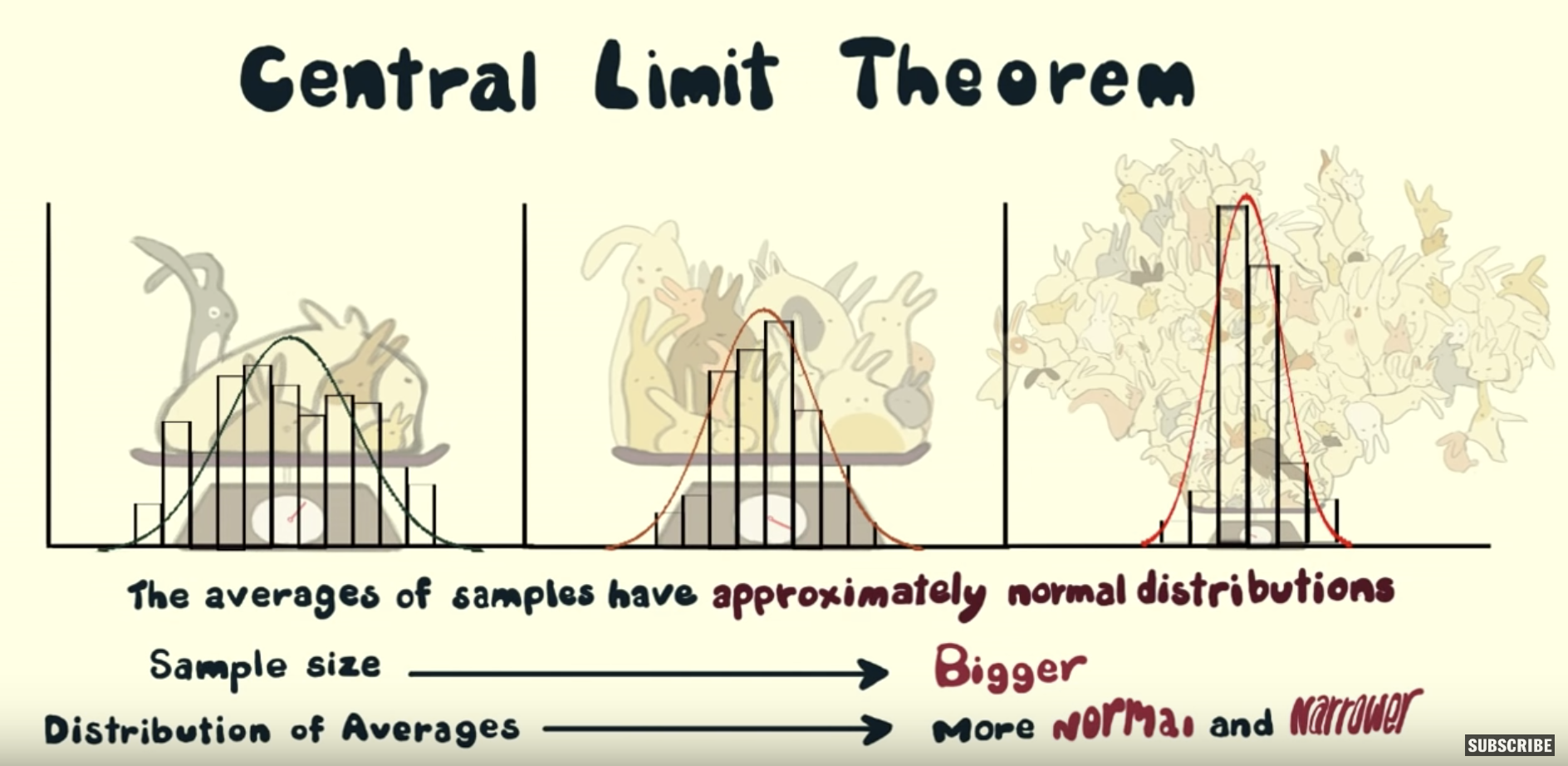## 7.5 Conclusion

### 7.5.1 Sampling scenarios

In this chapter, we performed both tactile and virtual sampling exercises to infer about an unknown proportion. We also presented a case study of sampling in real life with polls. In each case, we used the sample proportion $$\widehat{p}$$ to estimate the population proportion $$p$$. However, we are not just limited to scenarios related to proportions. In other words, we can use sampling to estimate other population parameters using other point estimates as well. We present four more such scenarios in Table 7.5.

TABLE 7.5: Scenarios of sampling for inference
Scenario Population parameter Notation Point estimate Symbol(s)
1 Population proportion $$p$$ Sample proportion $$\widehat{p}$$
2 Population mean $$\mu$$ Sample mean $$\overline{x}$$ or $$\widehat{\mu}$$
3 Difference in population proportions $$p_1 - p_2$$ Difference in sample proportions $$\widehat{p}_1 - \widehat{p}_2$$
4 Difference in population means $$\mu_1 - \mu_2$$ Difference in sample means $$\overline{x}_1 - \overline{x}_2$$
5 Population regression slope $$\beta_1$$ Fitted regression slope $$b_1$$ or $$\widehat{\beta}_1$$

In the rest of this book, we’ll cover all the remaining scenarios as follows:

• In Chapter 8, we’ll cover examples of statistical inference for
• Scenario 2: The mean age $$\mu$$ of all pennies in circulation in the US.
• Scenario 3: The difference $$p_1 - p_2$$ in the proportion of people who yawn when seeing someone else yawn first minus the proportion of people who yawn without seeing someone else yawn first. This is an example of two-sample inference.
• In Chapter 9, we’ll cover an example of statistical inference for
• Scenario 4: The difference $$\mu_1 - \mu_2$$ in mean IMDb ratings for action and romance movies. This is another example of two-sample inference.
• In Chapter 10, we’ll cover an example of statistical inference for regression by revisiting the regression models for teaching score as a function of various instructor demographic variables you saw in Chapters 5 and 6.
• Scenario 5: The slope $$\beta_1$$ of the population regression line.

### 7.5.2 Central Limit Theorem

What you visualized in Figures 7.12 and 7.14 and summarized in Tables 7.1 and 7.3 was a demonstration of a famous theorem, or mathematically proven truth, called the Central Limit Theorem. It loosely states that when sample means are based on larger and larger sample sizes, the sampling distribution of these sample means becomes both more and more normally shaped and more and more narrow.

In other words, their sampling distribution increasingly follows a normal distribution and the variation of these sampling distributions gets smaller, as quantified by their standard errors.

Shuyi Chiou, Casey Dunn, and Pathikrit Bhattacharyya created a 3-minute and 38-second video at https://youtu.be/jvoxEYmQHNM explaining this crucial statistical theorem using the average weight of wild bunny rabbits and the average wingspan of dragons as examples. Figure 7.17 shows a preview of this video.FIGURE 7.17: Preview of Central Limit Theorem video.

Note the term margin of error, which here is “plus or minus 2.1 percentage points.” Most polls won’t produce an estimate that’s perfectly right; there will always be a certain amount of error caused by sampling variation. The margin of error of plus or minus 2.1 percentage points is saying that a typical range of errors for polls of this type is about $$\pm$$ 2.1%, in words from about 2.1% too small to about 2.1% too big. We can restate this as the interval of $$[41\% - 2.1\%, 41\% + 2.1\%] = [37.9\%, 43.1\%]$$ (this notation indicates the interval contains all values between 37.9% and 43.1%, including the end points of 37.9% and 43.1%). We’ll see in the next chapter that such intervals are known as confidence intervals.